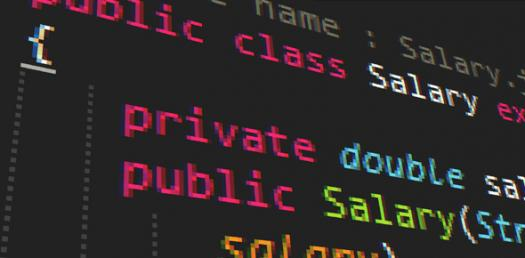Quiz No. 1 In Java Programming

20 QuestionsSettingsThe quiz is about the basic terminologies in Java programming. It also includes array declaration and the application of operators.

• 1.
Using the declaration below, what will be the final element of the array? int [ ] grades = new int;
• A.

• B.

• C.

• D.

Impossible to tell

• 2.
It is the Java keyword that creates inheritance.
• A.

Enlarge

• B.

Extends

• C.

Inherits

• D.

Inheritance

• 3.
It is the rules of a programming language.
• A.

Format

• B.

Logic

• C.

Object

• D.

Syntax

• 4.
It is the code/s inside a pair of curly braces.
• A.

Block

• B.

Brick

• C.

Function

• D.

Method

• 5.
• A.

10

• B.

11

• C.

12

• D.

13

• 6.
It is the characteristics of Java which ensures that a program can go only where it is designed to go and eliminates the possibility of altering system data unintentionally.
• A.

Java is simple.

• B.

Java is secure.

• C.

Java is free.

• D.

Java is portable.

• 7.
It is a form of Java program that runs locally on the command line.
• A.

Applets

• B.

Application

• C.

Midlets

• D.

Servlets

• 8.
It defines the common variables and methods of a set of objects.
• A.

Objects

• B.

Class

• C.

Function

• D.

Method

• 9.
It is the process of removing errors found in the program.
• A.

Editing

• B.

Debugging

• C.

Compiling

• D.

Running

• 10.
Which of the following is the data type used for a single character?
• A.

Byte

• B.

Short

• C.

Long

• D.

Char

• 11.
• A.

/ /

• B.

/* */

• C.

{ }

• D.

< >

• 12.
Which of the following is not a unary operator?
• A.

Negation

• B.

Decrement

• C.

Bitwise complement

• D.

Assignment

• 13.
Which of the following is not a Java keyword?
• A.

Default

• B.

For

• C.

Volatile

• D.

Of

• 14.
• A.

Byte

• B.

String

• C.

Char

• D.

Long

• 15.
Which of the following is an invalid first character of an identifier?
• A.

__

• B.

\$

• C.

A

• D.

8

• 16.
It is the length of the data type float.
• A.

8 bits

• B.

12 bits

• C.

16 bits

• D.

32 bits

• 17.
It is the length of the data type short.
• A.

8 bits

• B.

16 bits

• C.

32 bits

• D.

64 bits

• 18.
• A.

Int NumberOfStudents = 250;

• B.

Double Salary =0.0;

• C.

String NAME =

• D.

Char CivilStatus =

• 19.
It terminates every line of code in Java.
• A.

}

• B.

)

• C.

;

• D.

.

• 20.
It is the command used to compile Java program in the command prompt.
• A.

Ja

• B.

Jav

• C.

Java

• D.

Javac

Related Quizzes of Java Programming
Related Topics